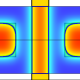## Learning category theory: a necessary evil?

The end of my last blog post (about isomorphism testing of reversible deterministic finite automata) explained how category theory gave me the idea that the simplified variant of my question about permutation group isomorphism should be easy to solve:

The idea to consider the case where the group is fixed came from reading an introduction to category theory, because those morphisms of left$M$-sets felt like cheating (or oversimplifying) to me. So I guessed that this case should be easy to solve, if I would only…

I hinted that this felt like cheating (or oversimplifying) to me, but I realized three month later that my original question could be reduced to the simplified variant by a brute force technique exploiting the fact that minimal generating sets of groups are very small. So it turned out that category theory was actually helpful and not cheating at all.

Why does a mathematician interested in logic, (semi-)lattice theory and universal algebra reads an introduction to category theory? Because it is a good readable (medium level) German book on a mathematical subject I’m currently interested in.And it is written by Martin Brandenburg, who always helped people like me, when they got stuck on questions related to category theory. If the explanations and examples in the book are as good as his contributions on MathOverflow and the MathStack Exchange site, then I would be fluent in both theory and practice after finishing that book.

But why am I interested in category theory?

• There are all those forgetful functors with left adjoints in universal algebra. And when doing semigroup theory, switching freely between monoids and semigroups by adjoining an identity is very common. Seems like a good idea to understand this.
• I like inverse semigroups, which are closely related to partial bijections, and hence to partial functions. Mathematicians don’t like partial functions, but category theory at least admits that each function has a domain and a codomain. Some existing work on partial functions has been phrased in the language of category theory.
• I have read basic introductions to category theory, but kept forgetting about adjoint functors. Then I worked two times thru a reasonable introduction to the adjoint functor theorem (by Chris Henderson), and hopefully settled this problem.
• I would like to better understand the Ext and Tor functors in group cohomology, and diagram chasing like the snake lemma.
• I like non-classical logic, including intuitionistic logic, linear logic and partition logic. My understanding of non-classical logic is mostly based on lattice theory. Sadly, lattice theory is a very confusing name, and it has only few followers. So if I translate my ideas about non-classical logic and lattice theory into the language of category theory, then I should be able to reach a more relevant audience.
• I liked assembly language when I started to program, and set theory feels similar to assembly language. Category theory is one alternative to get around all the engrained prejudices about logic and model theory embedded into mainstream ZFC set theory.

Maybe I could find more reasons, but let’s talk about the content now

• Category theorists seem to have a strange relation to calculations. The introduction to the adjoint functor theorem by Chris Henderson often has imbalanced parenthesis in the formulas (my printout has those marked on page 6, 7, 11, and 12), indicating that the formulas have at least some errors. Sometimes (like the$=\phi_{C,D}(\phi_{C,D}^{-1}(f)$ near the bottom of page 7) I couldn’t figure out what the correct formula was supposed to be.
• The book by Martin Brandenburg has fewer of those unnecessary errors, but it is still annoying if$f\in \text{Hom}(A,C)$ is written instead of$f\in \text{Hom}(A,B)$ when explaining the Yoneda construction on page 109, which is difficult for me even without such errors.
• I actually had to return the book while reading chapter 5 on the Yoneda construction a second time. I bought my own copy of the book in the meantime, but haven’t resumed reading yet.
• Category theorists are similar to physicists in not noticing the places where the computational complexity can explode. So they claim only the universal property would be important, and the concrete representation an unimportant detail. But identity testing for the free modular lattice on 4 generators is undecidable, it is NP-complete for the free Boolean algebra, and trivial for free abelian groups. The universal algebra people are much more aware of such niceties, something which can easily get lost when doing universal algebra through the lens of category theory.

My overall conclusion is that I learned quite a bit from working through the category theory book, but that it were the concrete examples from which I benefitted most. But I still would not really recommend learning category theory to somebody who doesn’t know yet why he wants to learn it.